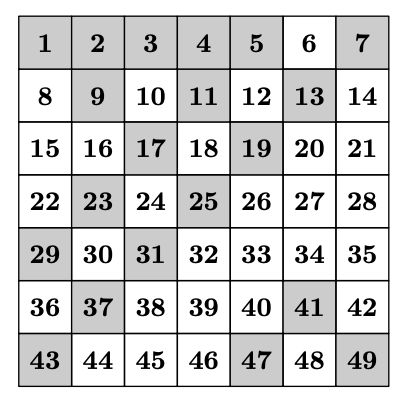시간 제한 메모리 제한 제출 정답 맞은 사람 정답 비율
1 초 512 MB 9 9 8 100.000%

## 문제

Inspired by the Ulam spiral, which unravels strange patterns of prime numbers' distribution, Petya decided to come up with his own analog.

Petya writes down integers from $1$ to $n^2$ into square table of size $n\times n$ starting from the upper left corner. Numbers from $1$ to $n$ go into the first row, numbers from $n + 1$ to $2n$ --- into the second row, and so on.

Then he colors cells which contain the number with no more than $k$ different divisors. After that, Petya studies the resulting picture in hope of finding some patterns. For example, if $n = 7$, $k = 3$, Petya will get the following picture:Help Petya, print the picture which he will get after coloring, representing colored cells with asterisks <<*>>, and non-colored cells with dots <<.>>.

## 입력

Input contains two integers $n$ and $k$ ($1 \le n \le 40$, $1 \le k \le n^2$).

## 출력

Output $n$ lines, each one containing $n$ characters. If the $j$-th cell of $i$-th row of Petya's table is colored, then $j$-th character of $i$-th line should be equal to <<*>>, and <<.>> otherwise.

## 예제 입력 1

7 3


## 예제 출력 1

*****.*
.*.*.*.
..*.*..
.*.*...
*.*....
.*...*.
*...*.*